# Low level API#

Introduction to Gammapy analysis using the low level API.

## Prerequisites#

• Understanding the gammapy data workflow, in particular what are DL3 events and instrument response functions (IRF).

• Understanding of the data reduction and modeling fitting process as shown in the analysis with the high level interface tutorial High level interface

## Context#

This notebook is an introduction to gammapy analysis this time using the lower level classes and functions the library. This allows to understand what happens during two main gammapy analysis steps, data reduction and modeling/fitting.

Objective: Create a 3D dataset of the Crab using the H.E.S.S. DL3 data release 1 and perform a simple model fitting of the Crab nebula using the lower level gammapy API.

## Proposed approach#

Here, we have to interact with the data archive (with the `DataStore`) to retrieve a list of selected observations (`Observations`). Then, we define the geometry of the `MapDataset` object we want to produce and the maker object that reduce an observation to a dataset.

We can then proceed with data reduction with a loop over all selected observations to produce datasets in the relevant geometry and stack them together (i.e.sum them all).

In practice, we have to:

## Setup#

First, we setup the analysis by performing required imports.

```from pathlib import Path
from astropy import units as u
from astropy.coordinates import SkyCoord
from regions import CircleSkyRegion

# %matplotlib inline
import matplotlib.pyplot as plt
from IPython.display import display
from gammapy.data import DataStore
from gammapy.datasets import MapDataset
from gammapy.estimators import FluxPointsEstimator
from gammapy.makers import FoVBackgroundMaker, MapDatasetMaker, SafeMaskMaker
from gammapy.maps import MapAxis, WcsGeom
from gammapy.modeling import Fit
from gammapy.modeling.models import (
FoVBackgroundModel,
PointSpatialModel,
PowerLawSpectralModel,
SkyModel,
)
from gammapy.utils.check import check_tutorials_setup
from gammapy.visualization import plot_npred_signal
```

## Check setup#

```check_tutorials_setup()
```
```System:

python_executable      : /home/runner/work/gammapy-docs/gammapy-docs/gammapy/.tox/build_docs/bin/python
python_version         : 3.9.18
machine                : x86_64
system                 : Linux

Gammapy package:

version                : 1.2.dev592+gfdc3f4b1f
path                   : /home/runner/work/gammapy-docs/gammapy-docs/gammapy/.tox/build_docs/lib/python3.9/site-packages/gammapy

Other packages:

numpy                  : 1.26.0
scipy                  : 1.11.3
astropy                : 5.2.2
regions                : 0.7
click                  : 8.1.7
yaml                   : 6.0.1
IPython                : 8.16.1
jupyterlab             : not installed
matplotlib             : 3.8.0
pandas                 : not installed
healpy                 : 1.16.5
iminuit                : 2.24.0
sherpa                 : 4.15.1
naima                  : 0.10.0
emcee                  : 3.1.4
corner                 : 2.2.2
ray                    : 2.7.0

Gammapy environment variables:

GAMMAPY_DATA           : /home/runner/work/gammapy-docs/gammapy-docs/gammapy-datasets/dev
```

## Defining the datastore and selecting observations#

We first use the `DataStore` object to access the observations we want to analyse. Here the H.E.S.S. DL3 DR1.

```data_store = DataStore.from_dir("\$GAMMAPY_DATA/hess-dl3-dr1")
```

We can now define an observation filter to select only the relevant observations. Here we use a cone search which we define with a python dict.

We then filter the `ObservationTable` with `select_observations`.

```selection = dict(
type="sky_circle",
frame="icrs",
lon="83.633 deg",
lat="22.014 deg",
)
selected_obs_table = data_store.obs_table.select_observations(selection)
```

We can now retrieve the relevant observations by passing their `obs_id` to the `get_observations` method.

```observations = data_store.get_observations(selected_obs_table["OBS_ID"])
```

## Preparing reduced datasets geometry#

Now we define a reference geometry for our analysis, We choose a WCS based geometry with a binsize of 0.02 deg and also define an energy axis:

```energy_axis = MapAxis.from_energy_bounds(1.0, 10.0, 4, unit="TeV")

geom = WcsGeom.create(
skydir=(83.633, 22.014),
binsz=0.02,
width=(2, 2),
frame="icrs",
proj="CAR",
axes=[energy_axis],
)

# Reduced IRFs are defined in true energy (i.e. not measured energy).
energy_axis_true = MapAxis.from_energy_bounds(
0.5, 20, 10, unit="TeV", name="energy_true"
)
```

Now we can define the target dataset with this geometry.

```stacked = MapDataset.create(
geom=geom, energy_axis_true=energy_axis_true, name="crab-stacked"
)
```

## Data reduction#

### Create the maker classes to be used#

The `MapDatasetMaker` object is initialized as well as the `SafeMaskMaker` that carries here a maximum offset selection.

```offset_max = 2.5 * u.deg
maker = MapDatasetMaker()
methods=["offset-max", "aeff-max"], offset_max=offset_max
)

circle = CircleSkyRegion(center=SkyCoord("83.63 deg", "22.14 deg"), radius=0.2 * u.deg)
```

### Perform the data reduction loop#

```for obs in observations:
# First a cutout of the target map is produced
cutout = stacked.cutout(
obs.get_pointing_icrs(obs.tmid), width=2 * offset_max, name=f"obs-{obs.obs_id}"
)
# A MapDataset is filled in this cutout geometry
dataset = maker.run(cutout, obs)
# The data quality cut is applied
# fit background model
dataset = maker_fov.run(dataset)
print(
f"Background norm obs {obs.obs_id}: {dataset.background_model.spectral_model.norm.value:.2f}"
)
# The resulting dataset cutout is stacked onto the final one
stacked.stack(dataset)

print(stacked)
```
```Background norm obs 23523: 0.99
Background norm obs 23526: 1.08
Background norm obs 23559: 0.99
Background norm obs 23592: 1.10
MapDataset
----------

Name                            : crab-stacked

Total counts                    : 2479
Total background counts         : 2112.97
Total excess counts             : 366.03

Predicted counts                : 2112.97
Predicted background counts     : 2112.97
Predicted excess counts         : nan

Exposure min                    : 3.75e+08 m2 s
Exposure max                    : 3.48e+09 m2 s

Number of total bins            : 40000
Number of fit bins              : 40000

Fit statistic type              : cash
Fit statistic value (-2 log(L)) : nan

Number of models                : 0
Number of parameters            : 0
Number of free parameters       : 0
```

### Inspect the reduced dataset#

```stacked.counts.sum_over_axes().smooth(0.05 * u.deg).plot(stretch="sqrt", add_cbar=True)
plt.show()
```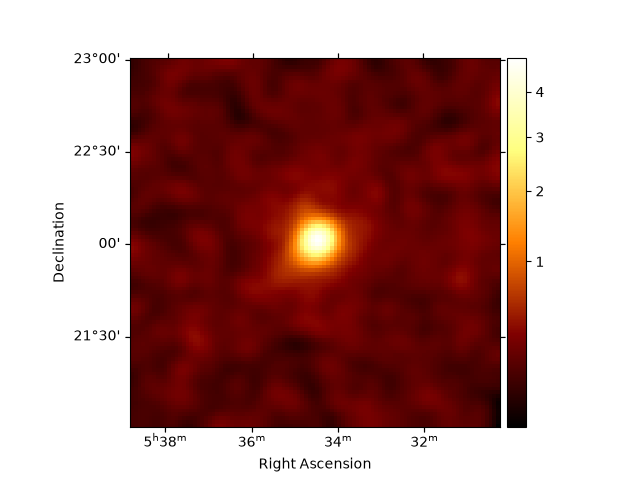## Save dataset to disk#

It is common to run the preparation step independent of the likelihood fit, because often the preparation of maps, PSF and energy dispersion is slow if you have a lot of data. We first create a folder:

```path = Path("analysis_2")
path.mkdir(exist_ok=True)
```

And then write the maps and IRFs to disk by calling the dedicated `write` method:

```filename = path / "crab-stacked-dataset.fits.gz"
stacked.write(filename, overwrite=True)
```

## Define the model#

We first define the model, a `SkyModel`, as the combination of a point source `SpatialModel` with a powerlaw `SpectralModel`:

```target_position = SkyCoord(ra=83.63308, dec=22.01450, unit="deg")
spatial_model = PointSpatialModel(
lon_0=target_position.ra, lat_0=target_position.dec, frame="icrs"
)

spectral_model = PowerLawSpectralModel(
index=2.702,
amplitude=4.712e-11 * u.Unit("1 / (cm2 s TeV)"),
reference=1 * u.TeV,
)

sky_model = SkyModel(
spatial_model=spatial_model, spectral_model=spectral_model, name="crab"
)

bkg_model = FoVBackgroundModel(dataset_name="crab-stacked")
```

Now we assign this model to our reduced dataset:

```stacked.models = [sky_model, bkg_model]
```

## Fit the model#

The `Fit` class is orchestrating the fit, connecting the `stats` method of the dataset to the minimizer. By default, it uses `iminuit`.

Its constructor takes a list of dataset as argument.

```fit = Fit(optimize_opts={"print_level": 1})
result = fit.run([stacked])
```

The `FitResult` contains information about the optimization and parameter error calculation.

```print(result)
```
```OptimizeResult

backend    : minuit
success    : True
message    : Optimization terminated successfully.
nfev       : 142
total stat : 16240.97

CovarianceResult

backend    : minuit
method     : hesse
success    : True
message    : Hesse terminated successfully.
```

The fitted parameters are visible from the `Models` object.

```print(stacked.models.to_parameters_table())
```
```     model         type      name     value    ...    max    frozen is_norm link
---------------- -------- --------- ---------- ... --------- ------ ------- ----
crab spectral     index 2.6000e+00 ...       nan  False   False
crab spectral amplitude 4.5911e-11 ...       nan  False    True
crab spectral reference 1.0000e+00 ...       nan   True   False
crab  spatial     lon_0 8.3619e+01 ...       nan  False   False
crab  spatial     lat_0 2.2024e+01 ... 9.000e+01  False   False
crab-stacked-bkg spectral      norm 9.3478e-01 ...       nan  False    True
crab-stacked-bkg spectral      tilt 0.0000e+00 ...       nan   True   False
crab-stacked-bkg spectral reference 1.0000e+00 ...       nan   True   False
```

Here we can plot the number of predicted counts for each model and for the background in our dataset. In order to do this, we can use the `plot_npred_signal` function.

```plot_npred_signal(stacked)
plt.show()
```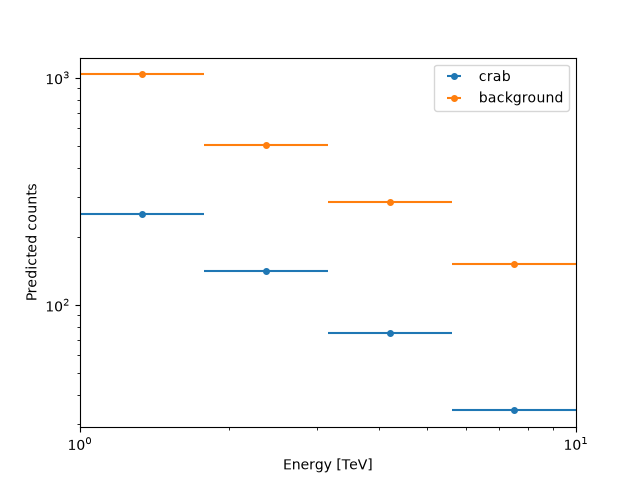### Inspecting residuals#

For any fit it is useful to inspect the residual images. We have a few options on the dataset object to handle this. First we can use `plot_residuals_spatial` to plot a residual image, summed over all energies:

```stacked.plot_residuals_spatial(method="diff/sqrt(model)", vmin=-0.5, vmax=0.5)
plt.show()
```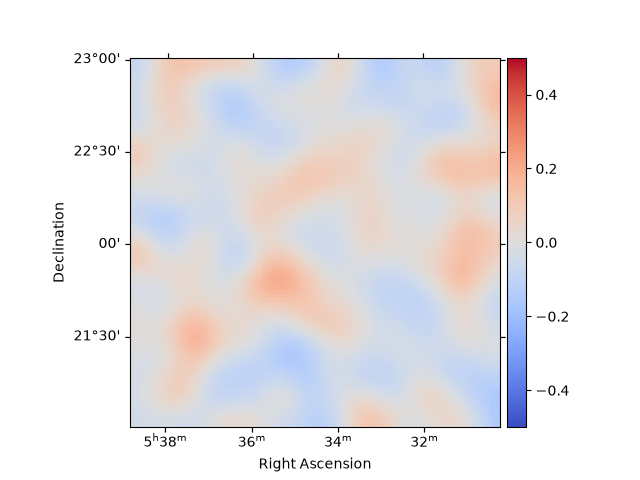In addition, we can also specify a region in the map to show the spectral residuals:

```region = CircleSkyRegion(center=SkyCoord("83.63 deg", "22.14 deg"), radius=0.5 * u.deg)

stacked.plot_residuals(
kwargs_spatial=dict(method="diff/sqrt(model)", vmin=-0.5, vmax=0.5),
kwargs_spectral=dict(region=region),
)
plt.show()
```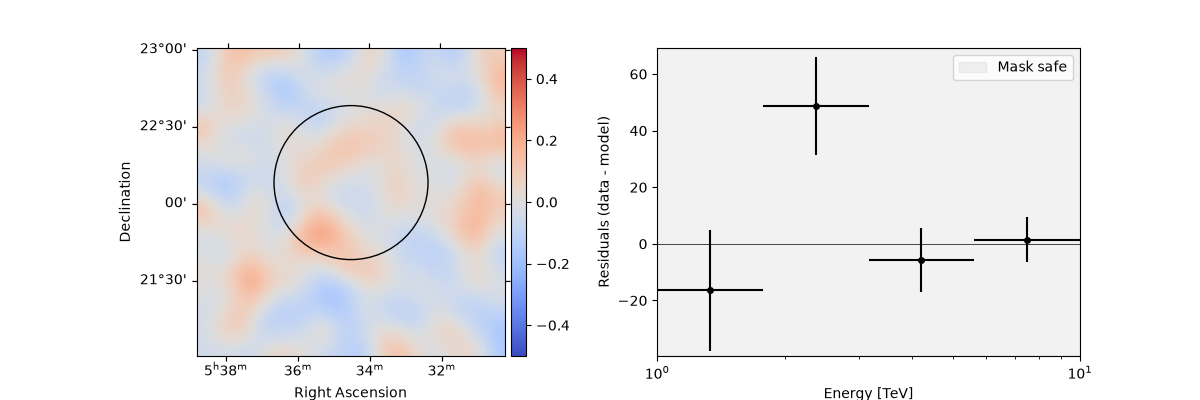We can also directly access the `.residuals()` to get a map, that we can plot interactively:

```residuals = stacked.residuals(method="diff")
residuals.smooth("0.08 deg").plot_interactive(
)
plt.show()
```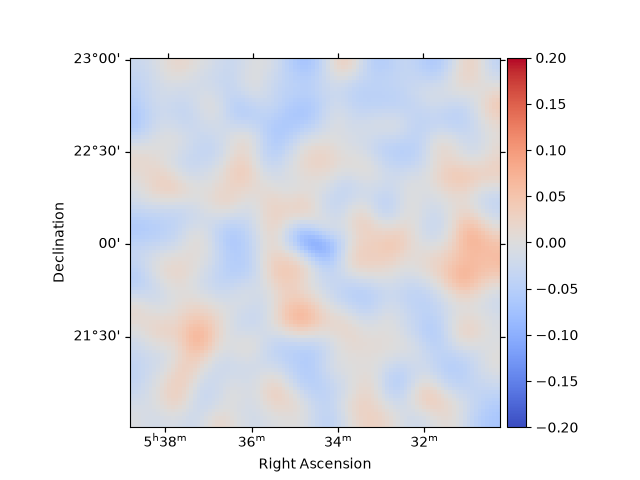```interactive(children=(SelectionSlider(continuous_update=False, description='Select energy:', layout=Layout(width='50%'), options=('1.00 TeV - 1.78 TeV', '1.78 TeV - 3.16 TeV', '3.16 TeV - 5.62 TeV', '5.62 TeV - 10.0 TeV'), style=SliderStyle(description_width='initial'), value='1.00 TeV - 1.78 TeV'), RadioButtons(description='Select stretch:', options=('linear', 'sqrt', 'log'), style=DescriptionStyle(description_width='initial'), value='linear'), Output()), _dom_classes=('widget-interact',))
```

## Plot the fitted spectrum#

### Making a butterfly plot#

The `SpectralModel` component can be used to produce a, so-called, butterfly plot showing the envelope of the model taking into account parameter uncertainties:

```spec = sky_model.spectral_model
```

Now we can actually do the plot using the `plot_error` method:

```energy_bounds = [1, 10] * u.TeV
spec.plot(energy_bounds=energy_bounds, energy_power=2)
ax = spec.plot_error(energy_bounds=energy_bounds, energy_power=2)
plt.show()
```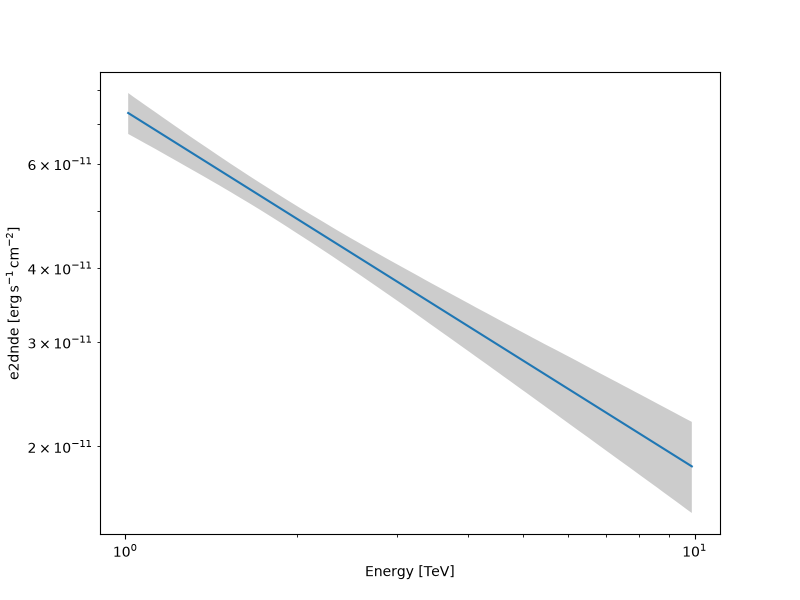### Computing flux points#

We can now compute some flux points using the `FluxPointsEstimator`.

Besides the list of datasets to use, we must provide it the energy intervals on which to compute flux points as well as the model component name.

```energy_edges = [1, 2, 4, 10] * u.TeV
fpe = FluxPointsEstimator(energy_edges=energy_edges, source="crab")
```
```flux_points = fpe.run(datasets=[stacked])

ax = spec.plot_error(energy_bounds=energy_bounds, energy_power=2)
flux_points.plot(ax=ax, energy_power=2)
plt.show()
```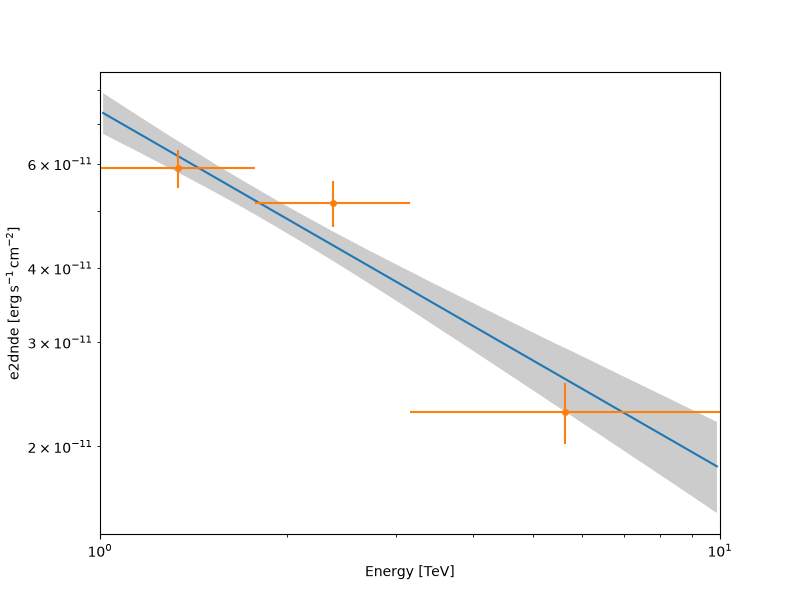Total running time of the script: ( 0 minutes 18.430 seconds)

Gallery generated by Sphinx-Gallery